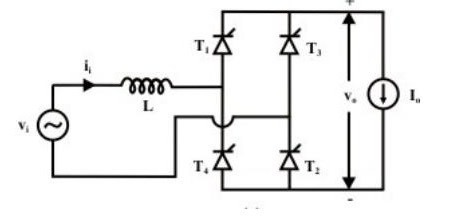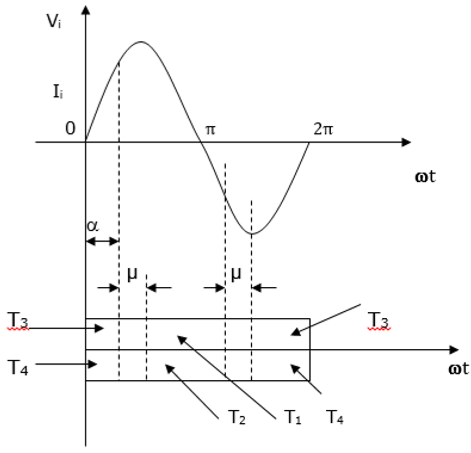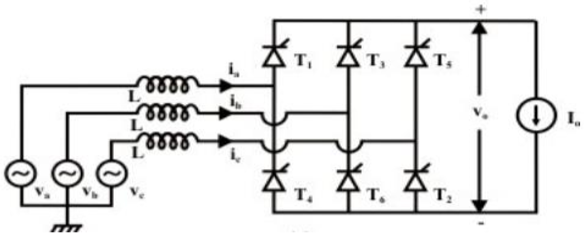# Effect of Source Inductance

The analysis of most converters is usually simplified under ideal conditions (no source impedance). However, this assumption is not justified since source impedance is normally inductive with a negligible resistive element.

Source inductance has a significant impact on the converter performance because its presence alters the output voltage of the converter. As a result, the output voltage reduces as the load current reduces. In addition, the input current and output voltage waveforms change significantly.

Source inductance effect on a converter is analyzed in the following two ways.

## Effect on Single Phase

Assuming that the converter operates in conduction mode and the ripple from the load current is negligible, the open circuit voltage becomes equal to average DC output at a firing angle of α.The diagram below shows a fully controlled converter with source in single phase. The thyristors T3 and T4 are assumed to be in conduction mode when t = 0. On the other hand, T1 and T2 fire when ωt = αWhere −

• Vi = input voltage
• Ii = input current
• Vo = output voltage
• Io = output voltage

When there is no source inductance, commutation will occur at T3 and T4. Immediately thyristors T1 and T2 are switched ON. This will lead the input polarity to change instantaneously. In the presence of source inductance, change of polarity and commutation does not occur instantaneously. Thus, T3 and T4 do not commutate as soon as T1 and T2 are switched ON.

At some interval, all the four thyristors will be conducting. This conducting interval is called the overlap interval (μ).

The overlap during commutation reduces the DC output voltage and the angle of extinction γ resulting in failed commutation when αis close to 180°. This is shown by the waveform below.## Effect on Three Phase

Just like the single-phase converter, there are no instantaneous commutations due to the presence of the source inductances. Taking the source inductances into consideration, the effects (qualitative) on the converter performance is the same as in a single phase converter. This is shown in the diagram below.